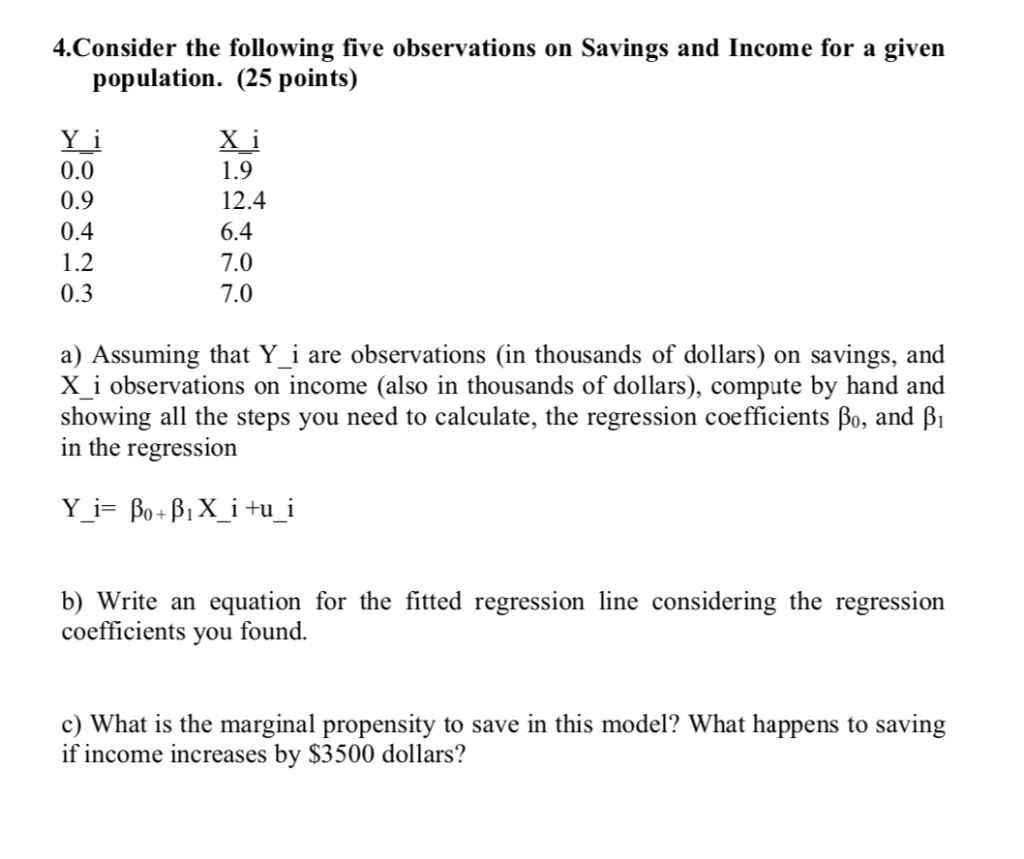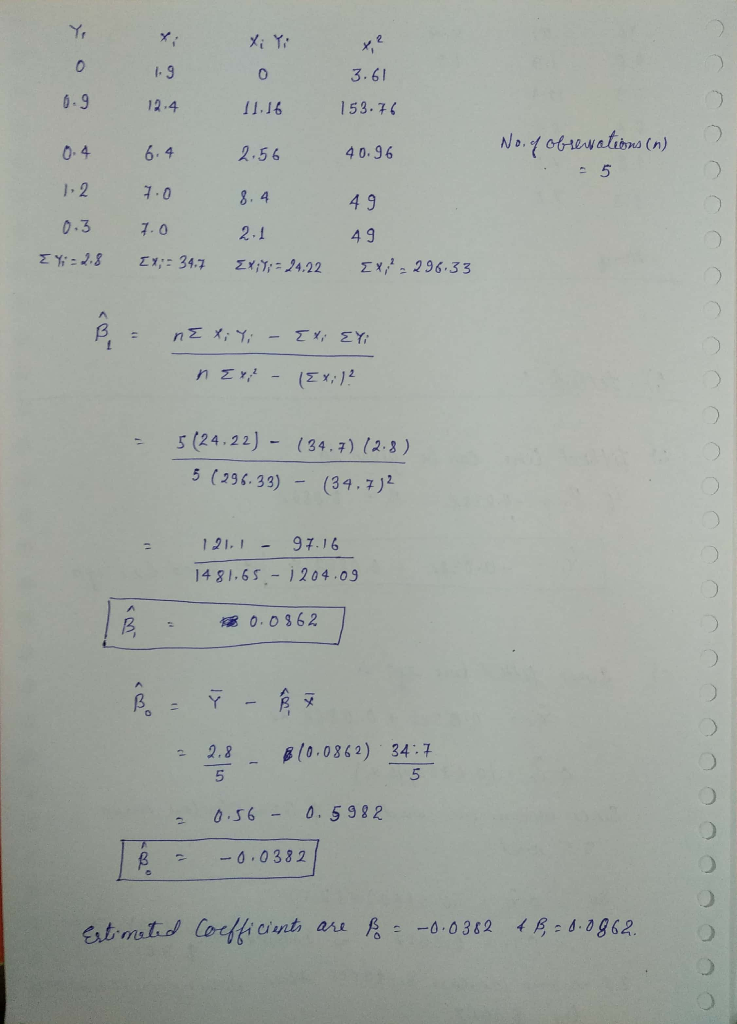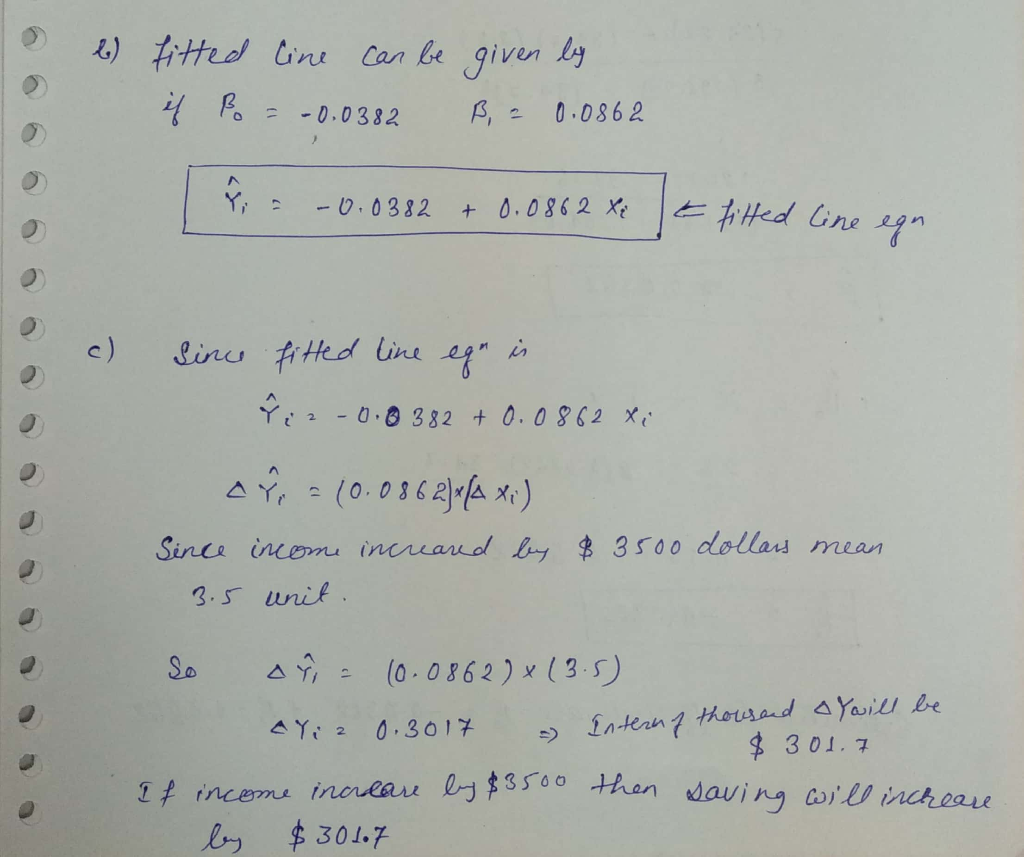# 4.Consider the following five observations on Savings and Income for a given population. (25 poin...4.Consider the following five observations on Savings and Income for a given population. (25 points) Y i 0.0 0.9 0.4 12.4 6.4 7.0 7.0 0.3 a) Assuming that Y_i are observations (in thousands of dollars) on savings, and X_i observations on income (also in thousands of dollars), compute by hand and showing all the steps you need to calculate, the regression coefficients β0, and β1 in the regression b) Write an equation for the fitted regression line considering the regression coefficients you found. c) What is the marginal propensity to save in this model? What happens to saving if income increases by S3500 dollars?##### Add Answer of: 4.Consider the following five observations on Savings and Income for a given population. (25 poin...
More Homework Help Questions Additional questions in this topic.

• #### This data given: GDP=\$110; Income earned by citizens abroad=\$5; income foreigners earn here=\$15; loss from depreciation=\$4; indirect business taxes=\$6; business subsidies=\$2; retained earnings=\$5; corporate income taxes=\$6; social insurance contributions=

Need Online Homework Help?